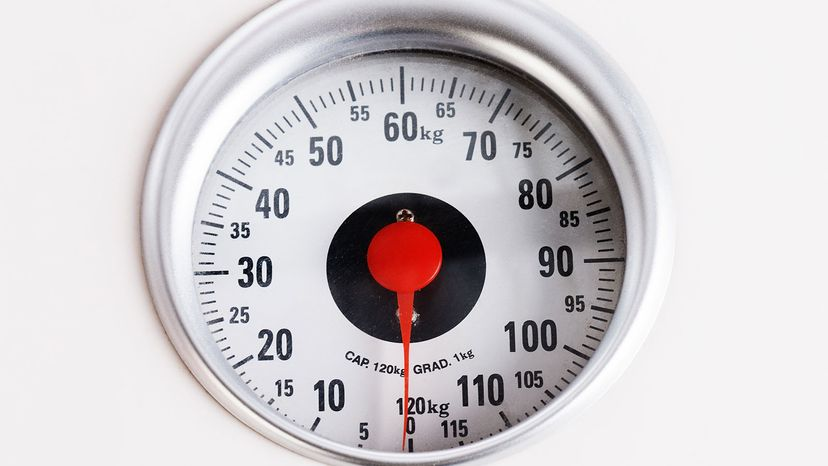# How to Convert Kilograms to Pounds

By: Mark Mancini  |It's simple to convert kilograms to pounds. RapidEye/Getty Images

In his unsuccessful bid for the presidency, former Rhode Island Gov. (and Sen.) Lincoln Chafee argued that the United States should embrace a different system of measurement. "Let's be bold—let's join the rest of the world and go metric," proclaimed Chafee at his 2015 campaign launch.

Some readers might recall the U.S. Metric Conversion Act of 1975. Signed into law by then-President Gerald Ford, it declared "a national policy of coordinating the increasing use of the metric system in the United States."

But there was a major caveat. To quote Ford himself, this legislation was "completely voluntary." Since it didn't require anyone to switch over to metric measurements, most of his countrymen didn't bother.

Still, the rest of the world (apart from a few other countries) uses metric so there's bound to be times you have to convert kilograms to pounds, and vice versa.

Pounds are a means of measuring weight. Devotees of the metric system use kilograms to measure a related — but distinct — phenomenon: mass.

In spite of this inconsistency, you often hear "kilograms" and "pounds" mentioned in the same breath. Today, we'll show you how to convert one into the other.

### Converting Kilograms to Pounds (The Textbook Way)

Here's an important figure to remember (if you can): 2.2046

See, 1 kilogram is equal to 2.2046 pounds. So, to make a conversion between the two measurements, you'll need to use this number.

Let's say your Canadian friend Bob tells you his dog Rex weighs 34 kilograms. What does that mean in terms of pounds?

Unfortunately, the formula isn't quite as simple as just taking the relevant amount of kilograms and multiplying it by 2.2046. But don't worry; it's still pretty darn simple.

First, we must write down two separate fractions: 34 kilograms divided by 1 and 2.2046 pounds divided by 1 kilogram. Then, we'll have to multiply the former by the latter.

Here's what that equation looks like when expressed numerically:

(34 kilograms/1) x (2.2046 pounds/1 kilogram)

The division by 1 might seem pointless. But we need it to do our conversion. Note that the "kilograms" notations in those two fractions will cancel each other out. But the "pounds" notation? That's not going anywhere. Ergo, we're left with:

(34/1) x (2.2046 pounds/1)

Like they taught us in grade school, a number divided by 1 equals itself. With this knowledge in mind, we can now rewrite our equation like so:

34 x 2.2046 pounds = 74.96 pounds

Therefore: 34 kilograms is equal to 74.96 pounds, or 75 pounds, rounded up.

Yeah, it's probably safe to assume Rex isn't lap dog material ...

### Converting Kilograms to Pounds (the Quick Way)

Let's say you're not in math class any more. You just want to know approximately how much Bob's darn dog weighs in pounds and right away.

Simply multiply 34 by 2.2. This gives you 74.8 pounds. Close enough.

Or if you have no calculator handy:

1. Multiply your kilograms by 2.
2. Take the total and move the decimal space one spot to the left.
3. Add the two numbers together.

Here's how it looks:

1. 34 x 2 = 68
2. Moving the decimal space one spot to the left gives you 6.8
3. 68 + 6.8 = 74.8

Here is a handy converter to turn kilograms into pounds:

### Converting Pounds to Kilograms

OK, having established Rex's weight in pounds, let's take the reverse approach. How do you convert pounds into kilograms?

The technique is similar; again, we'll be multiplying one fraction by another. Let's say Rex weighs 68 pounds.

Here's our new formula:

(68 pounds/1) x (1 kilogram/2.2046 pounds)

This time, the two "pounds" will be canceling each other out. We are now left with the following:

(68/1) x (1 kilogram/2.2046)

And hey, since we've got a number divided by one here, it's possible to simplify things a bit.

68 x (1 kilogram/2.2046)

Pause! Take a look at everything between those two parentheses. What's 1 kilogram divided by 2.2046?

Why 0.45 kilogram, of course. So now, we've got:

68 x 0.45 kilogram = 30.6 kilograms

The quick way? Just divide 68 by 2.2. You'll get 30.9 kilograms.